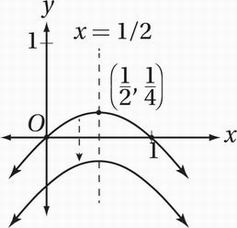# SAT Math Multiple Choice Question 640: Answer and Explanation

### Test Information

Question: 640

10. The function f is defined by the equation f(x) = x - x2. Which of the following represents a quadratic with no real zeros?

• A.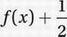• B.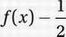• C.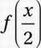• D.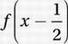Perhaps the simplest way to begin this problem is to draw a quick sketch of the function in the xy-plane, and then compare this graph to the transformations of the original function given in the choices. Notice that the original function f(x) = x - x2 is easily factored as f(x) = x (1 - x). The Zero Product Property (Chapter 9, Lesson 5) tells us that this function must have zeros at x = 0 and x = 1. Notice, also, that since the coefficient of the x2 term in the original function is negative (-1), the graph of this quadratic is an "open-down" parabola. Also, the axis of symmetry is halfway between the zeros, at x = ½. Plugging x = ½ back into the function gives us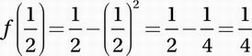, and therefore, the vertex of the parabola is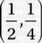.